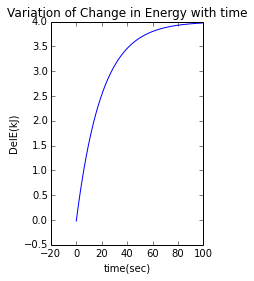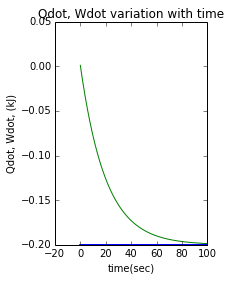# Chapter 3: Using Energy and the First Law of Thermodynamics

## Example 3.01, page: 39

In :
from __future__ import division
import math

#  Initialization  of  Variable
V1=0.1; # initial Volume m3
V2=0.2; # final volume in m3
P1=3.0; #pressure in bar
n1=1.5;
n2=1.0;
n3=0.0;

#calculations:
#pressure at stage 2
P2=P1*(V1/V2)**n1
# work done
W=(P2*V2-P1*V1)/(1-n1)
W2=P1*V1*math.log(V2/V1)
W3=P1*(V2-V1)

#Results
print  "a)work done = ", round(W*100,2),"kj"
print  "b)work done = ", round(W2*100,2),"kj"
print  "c)work done = ", round(W3*100,2),"kj"

a)work done =  17.57 kj
b)work done =  20.79 kj
c)work done =  30.0 kj


## Example 3.02, page: 45

In :
from __future__ import division
import math

# Initialization  of  Variable
delU = -4.6 #u2-u1;
W = 17.6 #work  done
m = 4    #mass

# calculations
# net heat transfer for the process
Q = W+m*delU;

#Results
print "Energy  transferred ", round(Q,2),"kJ"

Energy  transferred  -0.8 kJ


## Example 3.03, page: 46

In :
from __future__ import division
import math

#  Initialization  of  Variable
patm = 14.7 # atm pressure in  lbf/in**2
mpiston=100; # mass of piston in lb
g=32.2; # acc of gravity in ft/s2
A=1    #area
mair=0.6; # mass of air in lb
delu=18; # change in internal Energy in Btu/lb
k=1.6 #V2-V1 in ft3;

#calculations:
# pressure
P = mpiston*g/A/32.2/144+14.7
#work done
W = P*k*144/778
# the heat transfer from the resistor to the air
Q = W+mair*delu
# work done
W2 = patm*k*144/778
# elevation change
delz = k/A
# change in potential energy
PE = mpiston*g*delz/32.2/778
#the new heat transfer from the resistor to the air
Q2 = W2+PE+mair*delu

#Results
print  "a) Heat  transferred = ", round(Q,1),"Btu"
print  "b) Work  done = ", round(W2,2),"Btu"
print  "b) Heat  transferred = ", round (Q2, 1),"Btu"

a) Heat  transferred =  15.4 Btu
b) Work  done =  4.35 Btu
b) Heat  transferred =  15.4 Btu


## Example 3.04, page: 48

In :
from __future__ import division
import math

#  Initialization  of  Variable
h = -0.171; # constant in kW/m2
A = 1; # area in m2
Tb = 300 #temperature in K
Tf = 293 #temperature in K
W1dot = -60.0 # power in kW

#calculations:
# rate of energy transfer by heat
Qdot = h*A*(Tb-Tf)
# the power delivered through the output shaft
W2dot = Qdot-W1dot

#Results
print  "the  rate  of  heat  transfer = ", round(Qdot,1),"kW"
print  "the power delivered through the output shaft = ", round(W2dot,1),"kW"

the  rate  of  heat  transfer =  -1.2 kW
the power delivered through the output shaft =  58.8 kW


## Example 3.05, page: 50

In :
from __future__ import division
import math

#  Initialization  of  Variable
Wdot=-0.225; #electrical power input in W
a=5e-3; # side in m
h=150; # constant specific heat in W/m2
Tf=293#temperature in K

#calculations:
# area
A = a**2
#surface temperature of the chip at steady state
Tb=-Wdot/h/A+Tf;

#Results
print  "surface temperature of the chip at steady state = ", round(Tb,1),"kelvin"

surface temperature of the chip at steady state =  353.0 kelvin


## Example 3.06, page: 51

In :
from __future__ import division
import math
#from pylab import *
%pylab inline

#  Initialization  of  Variable
tau = 18; # torque in N.m
omega = 100; # frequency in rad/s
Wdotelec = -2.0; #electric power input in kW

#calculations:
#power associated with the rotating shaft
Wdotshaft = tau*omega/1000;
#power associated with the electricity flow
Wdot = Wdotelec+Wdotshaft;

#creating empty lists for plotting
t =[]
Edot =[]
Qdot = []
wdot = []
delE = []

for h in range(1000):
t.append((h-1)/10)
k=(h-1)/10
#energy rate balance
Edot.append(0.2*math.e**-(0.05*k))
wdot.append(Wdot)
Qdot.append(0.2*math.e**-(0.05*k) + Wdot)
# change in Energy
delE.append(4*(1-math.e**-(0.05*k)))

# plots
fig  = plt.figure()
ax = fig.add_subplot(1, 2, 1)
ax.plot(t,delE,'-')
xlabel('time(sec)')
ylabel('DelE(kJ)')
title('Variation of Change in Energy with time')
show()

fig  = plt.figure()
ax = fig.add_subplot(1, 2, 1)
ax.plot(t,wdot,'.')
ax.plot(t,Qdot,'-')
xlabel('time(sec)')
ylabel('Qdot, Wdot, (kJ)')
title('Qdot, Wdot variation with time')
show()

Populating the interactive namespace from numpy and matplotlib